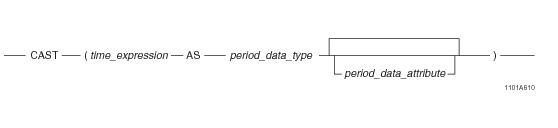# 15.00 - TIME-to-Period Conversion - Teradata Database

## Teradata Database SQL Functions, Operators, Expressions, and Predicates

Product
Release Number
15.00
Content Type
Programming Reference
Publication ID
B035-1145-015K
Language
English (United States)

## TIME-to-Period Conversion

### Purpose

Converts TIME data as PERIOD(TIME[(n)] [WITH TIME ZONE]) or PERIOD(TIMESTAMP[(n)][WITH TIME ZONE]).

### CAST Syntaxwhere:

 Syntax element … Specifies … time_expression the TIME data expression to be converted. period_data_type the target Period type to which time_expression is to be converted. period_data_attribute one of the following optional data attributes: FORMAT NAMED TITLE

### ANSI Compliance

This is ANSI SQL:2011 compliant.

As an extension to ANSI, CAST permits the use of data attribute phrases.

### Usage Notes

A TIME(n) [WITH TIME ZONE] value can be cast as PERIOD(TIME[(n)] [WITH TIME ZONE]) or PERIOD(TIMESTAMP[(n)] [WITH TIME ZONE]) using the CAST function.

If the target precision is higher than the source precision, trailing zeros are added in the result bounds to adjust the precision. If the target precision is lower than the source precision, an error is reported.

If the TIME source value contains leap seconds, the seconds portion gets adjusted to 59.999999 with the precision truncated to the target precision.

If the target type is PERIOD(TIME[(n)] [WITH TIME ZONE]), the result beginning element is set to the source value (in UTC). If the target type is PERIOD(TIMESTAMP[(n)] [WITH TIME ZONE]), the source time value get adjusted with respect to the current session time zone displacement from the corresponding UTC value; the date portion in the result beginning element is set to CURRENT_DATE, the time portion is set to the source value obtained after the above adjustment, and the resulting timestamp value is converted to UTC. If both the source and target are WITH TIME ZONE, the time zone field of the result beginning element is set to the source time zone field. If only the target has WITH TIME ZONE, the time zone field of the result beginning element is set to the current session time zone displacement. The result ending element is set to the result beginning bound plus one granule of the target type. If the result ending bound has a lower value than the result beginning bound for a target type of PERIOD(TIME[(n)] [WITH TIME ZONE) or the result ending element value exceeds the maximum corresponding TIMESTAMP value for a target type of PERIOD(TIMESTAMP[(n)] [WITH TIME ZONE), an error is reported.

Note: If the target type is WITH TIME ZONE, the result beginning and ending bounds have the same time zones.

Also, note that the result has the same value for the beginning bound and last value.

### Example

Assume pt is a TIME(0) column in table t with a value of TIME '12:12:12' and the current session time zone displacement is INTERVAL -'08:00' HOUR TO MINUTE.

In the following example, a TIME(0) column is cast as PERIOD(TIME(4) WITH TIME ZONE). The result beginning bound is formed form the source (in UTC) with trailing zeros added to make the precision 4 and the current session time zone displacement. The result ending element is set to the result beginning bound plus INTERVAL '0.0001' SECOND.

Note: The time zones of the result beginning and ending elements are the same.

`   SELECT CAST(pt AS PERIOD(TIME(4) WITH TIME ZONE)) FROM t;`

Returns a PERIOD(TIME(4) WITH TIME ZONE) value as follows:

`   ('12:12:12.0000-08:00', '12:12:12.0001-08:00')`

### Related Topics

For details on data types and data attributes, see SQL Data Types and Literals.